# Multiplication Tables

## NUMBERS AND OPERATIONSThe multiplication tables constitute one of the fundamental bases of Mathematics together with the operations of addition and subtraction.

They are an educational tool that facilitates the process of learning and memorizing multiplication from 2nd grade of Primary Education (7 years) through the orderly configuration (and from lowest to highest) of a set of diagrams where the values resulting from the multiplications are shown (for example, 3 x 5 = 15).

Thanks to their learning, mental arithmetic is empowered among the students, serving as a basis to learn multiplication and division successfully.

It is important that, rather than giving the students the multiplication tables so that they can learn them by heart, we explain that multiplication is nothing more than the successive sum of the same number and that it is very useful for quick and effortless accounts:

"Multiplication is just a shortcut to get large additions faster"

Only when children understand the usefulness of the multiplication algorithm will they make sense of learning the multiplication tables.

One last tip, it is essential to start by learning the easiest tables (for example the multiplication tables of 1, 2, 5 and 10) to later increase the level difficulty and avoid frustration in their learning among boys and girls.

MULTIPLY TABLES FROM 1 TO 10

We have prepared a template with the multiplication tables from 1 to 10 ready to download and print so that you can consult them when you need it and help you learn them quickly.

PYTHAGORAS MULTIPLY TABLES

We have also prepared a complete Pythagorean table where the multiplication tables from 1 to 10 are represented to facilitating very visual learning using patterns and symmetries and stimulate logical reasoning among students.

#### Memorize and learn 1 Times Multiplication Table

The multiplication Table of 1 is perfect for students to understand the essence of the multiplication algorithm, which is nothing more than the repeated addition of the same number so many times as indicated by your multiplier.

We can see that the Table of 1 is very easy to understand because the result (product) will always be equal to the multiplier digit. In other words, the result will always be the same number that we multiply by 1.

SPANISHENGLISH1 x 0 = 0

1 x 1 = 1 = 1

1 x 2 = 1 + 1 = 2

1 x 3 = 1 + 1 + 1 = 3

1 x 4 = 1 + 1 + 1 + 1 = 4

1 x 5 = 1 + 1 + 1 + 1 + 1 = 5

1 x 6 = 1 + 1 + 1 + 1 + 1 + 1 = 6

1 x 7 = 1 + 1 + 1 + 1 + 1 + 1 + 1 = 7

1 x 8 = 1 + 1 + 1 + 1 + 1 + 1 + 1 + 1 = 8

1 x 9 = 1 + 1 + 1 + 1 + 1 + 1 + 1 + 1 + 1 = 9

1 x 10 = 1 + 1 + 1 + 1 + 1 + 1 + 1 + 1 + 1 + 1 = 10#### Memorize and learn 2 Times Multiplication Table

The multiplication Table of 2 allows children to finish understanding what multiplication consists of since each result will be the result of adding the same number twice.

A strategy that we can use to facilitate their learning is that regardless of the multiplier digit, the result (product) of the multiplication will always be an even number (for example, 2 x 4 = 8).

We can also introduce the concept of "double a number" throughout the learning of the table of 2 since the result of each multiplication will always be double the digit of the multiplier (for example, 2 x 6 = 12, where 12 equals two times 6).

Another very simple trick that we can use to correctly learn the table of 2 is to add two ones to the result of the previous multiplication successively.

SPANISHENGLISH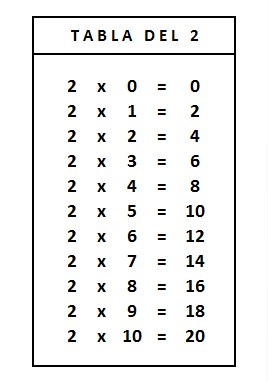2 x 0 = 0

2 x 1 = 2 = 2

2 x 2 = 2 + 2 = 4

2 x 3 = 2 + 2 + 2 = 6

2 x 4 = 2 + 2 + 2 + 2 = 8

2 x 5 = 2 + 2 + 2 + 2 + 2 = 10

2 x 6 = 2 + 2 + 2 + 2 + 2 + 2 = 12

2 x 7 = 2 + 2 + 2 + 2 + 2 + 2 + 2 = 14

2 x 8 = 2 + 2 + 2 + 2 + 2 + 2 + 2 + 2 = 16

2 x 9 = 2 + 2 + 2 + 2 + 2 + 2 + 2 + 2 + 2 = 18

2 x 10 = 2 + 2 + 2 + 2 + 2 + 2 + 2 + 2 + 2 + 2 = 20#### Memorize and learn 3 Times Multiplication Table

The multiplication Table of 3 is equivalent to adding the same number 3 times repeatedly.

We can also introduce the concept of "triple a number" throughout the learning of the table of 3 since the result of each multiplication will always be the triple of the multiplier digit (for example, 3 x 5 = 15, where 15 equals three times 5).

A great trick that we can use to successfully learn the 3 times table is to add three ones to the result of the previous multiplication successively, that is, 3 by 3.

SPANISHENGLISH3 x 0 = 0

3 x 1 = 3 = 3

3 x 2 = 3 + 3 = 6

3 x 3 = 3 + 3 + 3 = 9

3 x 4 = 3 + 3 + 3 + 3 = 12

3 x 5 = 3 + 3 + 3 + 3 + 3 = 15

3 x 6 = 3 + 3 + 3 + 3 + 3 + 3 = 18

3 x 7 = 3 + 3 + 3 + 3 + 3 + 3 + 3 = 21

3 x 8 = 3 + 3 + 3 + 3 + 3 + 3 + 3 + 3 = 24

3 x 9 = 3 + 3 + 3 + 3 + 3 + 3 + 3 + 3 + 3 = 27

3 x 10 = 3 + 3 + 3 + 3 + 3 + 3 + 3 + 3 + 3 + 3 = 30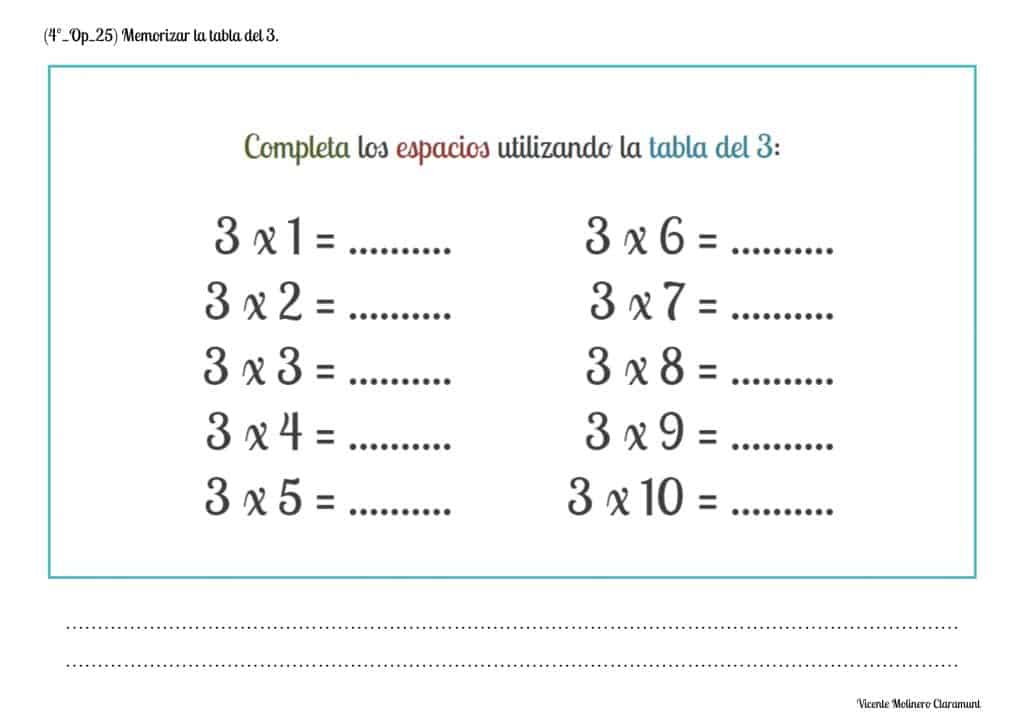#### Memorize and learn 4 Times Multiplication Table

The multiplication Table of 4 is to add the same number 4 times consecutively.

A strategy that we can use to facilitate their learning is that regardless of the multiplier digit, the result (product) of the multiplication will always end in 0 or an even number: 0 , 2, 4, 6 or 8.

In addition, we can facilitate the concept of "quadruple of a number" throughout the learning of the table of 4 since the result of each multiplication will always be the quadruple of the multiplier digit (for example, 4 x 6 = 24, where 24 equals four times 6).

A fun trick that we can use to quickly learn the 4 times table is to add four ones to the result of the previous multiplication in succession, that is, 4 by 4.

SPANISHENGLISH4 x 0 = 0

4 x 1 = 4 = 4

4 x 2 = 4 + 4 = 8

4 x 3 = 4 + 4 + 4 = 12

4 x 4 = 4 + 4 + 4 + 4 = 16

4 x 5 = 4 + 4 + 4 + 4 + 4 = 20

4 x 6 = 4 + 4 + 4 + 4 + 4 + 4 = 24

4 x 7 = 4 + 4 + 4 + 4 + 4 + 4 + 4 = 28

4 x 8 = 4 + 4 + 4 + 4 + 4 + 4 + 4 + 4 = 32

4 x 9 = 4 + 4 + 4 + 4 + 4 + 4 + 4 + 4 + 4 = 36

4 x 10 = 4 + 4 + 4 + 4 + 4 + 4 + 4 + 4 + 4 + 4 = 40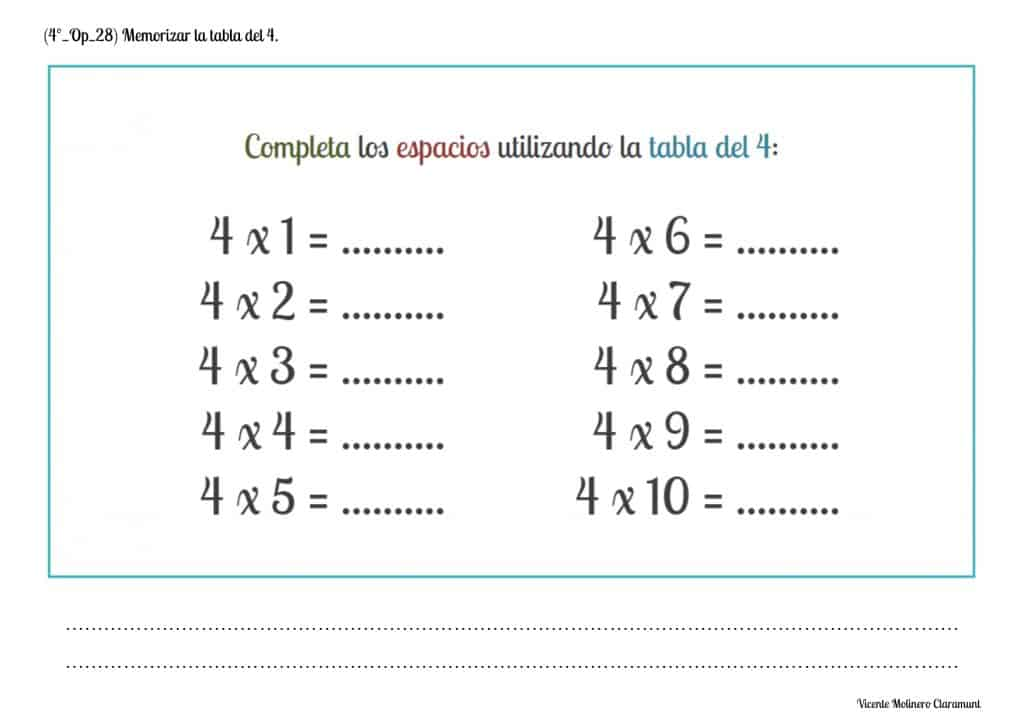#### Memorize and learn 5 Times Multiplication Table

The multiplication Table of 5 is one of the easiest to learn (along with the tables of 2 and 10) since the products are increased by 5 by 5 and therefore, the result will always end in 0 or 5 in the ones digit.

We can take advantage of their learning to introduce the concept of "quintuple of a number" throughout the learning of the table of 5 since the result of each multiplication will always be the five times of the multiplier digit, that is, quintuple the same number (for example, 5 x 7 = 35, where 35 equals five times 7).

A little trick that we can use to effectively learn the 5 times table is to add five ones to the result of the previous multiplication in succession, that is, 5 by 5.

SPANISHENGLISH5 x 0 = 0

5 x 1 = 5 = 5

5 x 2 = 5 + 5 = 10

5 x 3 = 5 + 5 + 5 = 15

5 x 4 = 5 + 5 + 5 + 5 = 20

5 x 5 = 5 + 5 + 5 + 5 + 5 = 25

5 x 6 = 5 + 5 + 5 + 5 + 5 + 5 = 30

5 x 7 = 5 + 5 + 5 + 5 + 5 + 5 + 5 = 35

5 x 8 = 5 + 5 + 5 + 5 + 5 + 5 + 5 + 5 = 40

5 x 9 = 5 + 5 + 5 + 5 + 5 + 5 + 5 + 5 + 5 = 45

5 x 10 = 5 + 5 + 5 + 5 + 5 + 5 + 5 + 5 + 5 + 5 = 50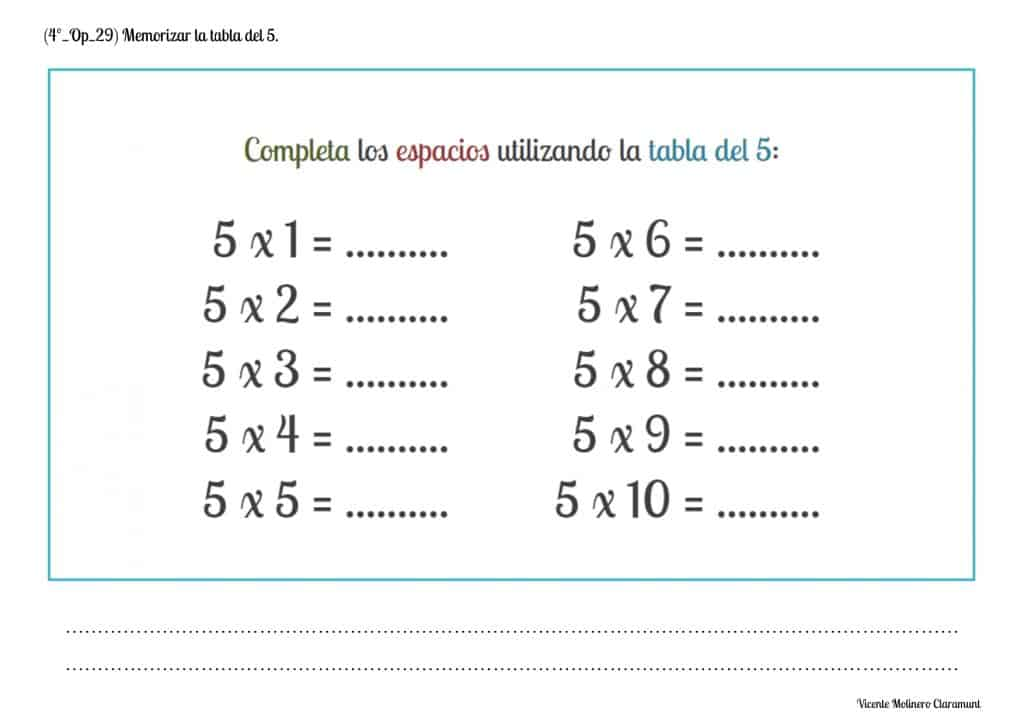#### Memorize and learn 6 Times Multiplication Table

The multiplication Table of 6 is equivalent to adding the same number 6 times in an echelon way.

A simple trick that we can use to facilitate their learning is that regardless of the multiplier digit, the result (product) of the multiplication will always end in 0 or a multiple of 2: 0, 2, 4, 6 or 8.

In addition, we can facilitate the concept of "sextuple of a number" throughout the learning of the table of 6 since the result of each multiplication will always be the sextuple of the multiplier digit (for example, 6 x 8 = 48, where 48 equals six times 8).

A basic strategy that we can use to learn the table of 6 is to add six ones to the result of the previous multiplication in succession, that is, 6 by 6.

SPANISHENGLISH6 x 0 = 0

6 x 1 = 6 = 6

6 x 2 = 6 + 6 = 12

6 x 3 = 6 + 6 + 6 = 18

6 x 4 = 6 + 6 + 6 + 6 = 24

6 x 5 = 6 + 6 + 6 + 6 + 6 = 30

6 x 6 = 6 + 6 + 6 + 6 + 6 + 6 = 36

6 x 7 = 6 + 6 + 6 + 6 + 6 + 6 + 6 = 42

6 x 8 = 6 + 6 + 6 + 6 + 6 + 6 + 6 + 6 = 48

6 x 9 = 6 + 6 + 6 + 6 + 6 + 6 + 6 + 6 + 6 = 54

6 x 10 = 6 + 6 + 6 + 6 + 6 + 6 + 6 + 6 + 6 + 6 = 60#### Memorize and learn 7 Times Multiplication Table

The multiplication Table of 7 means adding the same number 7 times consecutively.

The table of 7 and 8 are the most difficult to learn due to the size of the digit to be added (from 7 to 7) and the little relationship between their products since both even results can be given as odd and, therefore, there is no rule or pattern that enables their learning.

On this occasion, we can also facilitate the concept of "septuple of a number" throughout the learning of the table of 7 since the result of each multiplication will always be the septuble of the digit of the multiplier (for example, 7 x 8 = 56, where 56 equals seven times 8).

A useful strategy that we can use to learn the 7 times table is to add seven ones to the result of the previous multiplication progressively, that is, 7 by 7.

SPANISHENGLISH7 x 0 = 0

7 x 1 = 7 = 7

7 x 2 = 7 + 7 = 14

7 x 3 = 7 + 7 + 7 = 21

7 x 4 = 7 + 7 + 7 + 7 = 28

7 x 5 = 7 + 7 + 7 + 7 + 7 = 35

7 x 6 = 7 + 7 + 7 + 7 + 7 + 7 = 42

7 x 7 = 7 + 7 + 7 + 7 + 7 + 7 + 7 = 49

7 x 8 = 7 + 7 + 7 + 7 + 7 + 7 + 7 + 7 = 56

7 x 9 = 7 + 7 + 7 + 7 + 7 + 7 + 7 + 7 + 7 = 63

7 x 10 = 7 + 7 + 7 + 7 + 7 + 7 + 7 + 7 + 7 + 7 = 70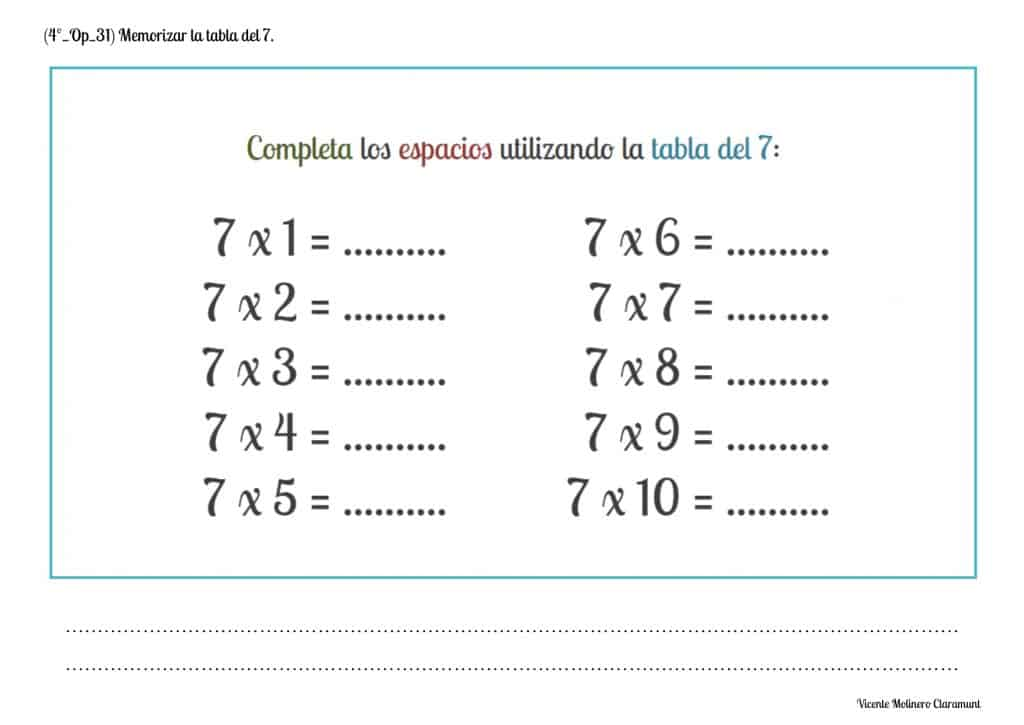#### Memorize and learn 8 Times Multiplication Table

The multiplication Table of 8 is to add the same number 8 times in an echelon way.

A cognitive strategy that we can use to facilitate their learning is that regardless of the multiplier digit, the result (product) of the multiplication will always end in 0 or a multiple of 2: 0, 2, 4, 6 or 8.

We can introduce with the table of 8 the concept of "octuple of a number" since the result of each multiplication will always be the octuple of the multiplier digit (for example, 8 x 9 = 72, where 72 equals eight times 9).

An interesting trick that we can use to learn the 8 times table is to add eight ones to the result of the previous multiplication progressively, that is, 8 by 8.

SPANISHENGLISH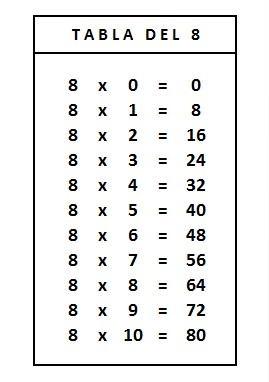8 x 0 = 0

8 x 1 = 8 = 8

8 x 2 = 8 + 8 = 16

8 x 3 = 8 + 8 + 8 = 24

8 x 4 = 8 + 8 + 8 + 8 = 32

8 x 5 = 8 + 8 + 8 + 8 + 8 = 40

8 x 6 = 8 + 8 + 8 + 8 + 8 + 8 = 48

8 x 7 = 8 + 8 + 8 + 8 + 8 + 8 + 8 = 56

8 x 8 = 8 + 8 + 8 + 8 + 8 + 8 + 8 + 8 = 64

8 x 9 = 8 + 8 + 8 + 8 + 8 + 8 + 8 + 8 + 8 = 72

8 x 10 = 8 + 8 + 8 + 8 + 8 + 8 + 8 + 8 + 8 + 8 = 80#### Memorize and learn 9 Times Multiplication Table

The multiplication Table of 9 lies in adding the same number 9 times consecutively.

On this occasion we can also introduce the concept of "nonuple of a number" throughout the learning of the table of 9 since the result of each multiplication will always be nine times of the multiplier digit, that is, nonuple the same number (for example, 9 x 3 = 27, where 27 equals nine times 3).

An entertaining trick that we can use to learn the 9 times table step by step is to add nine ones to the result of the previous multiplication progressively, that is, 9 by 9 .

SPANISHENGLISH9 x 0 = 0

9 x 1 = 9 = 9

9 x 2 = 9 + 9 = 18

9 x 3 = 9 + 9 + 9 = 27

9 x 4 = 9 + 9 + 9 + 9 = 36

9 x 5 = 9 + 9 + 9 + 9 + 9 = 45

9 x 6 = 9 + 9 + 9 + 9 + 9 + 9 = 54

9 x 7 = 9 + 9 + 9 + 9 + 9 + 9 + 9 = 63

9 x 8 = 9 + 9 + 9 + 9 + 9 + 9 + 9 + 9 = 72

9 x 9 = 9 + 9 + 9 + 9 + 9 + 9 + 9 + 9 + 9 = 81

9 x 10 = 9 + 9 + 9 + 9 + 9 + 9 + 9 + 9 + 9 + 9 = 90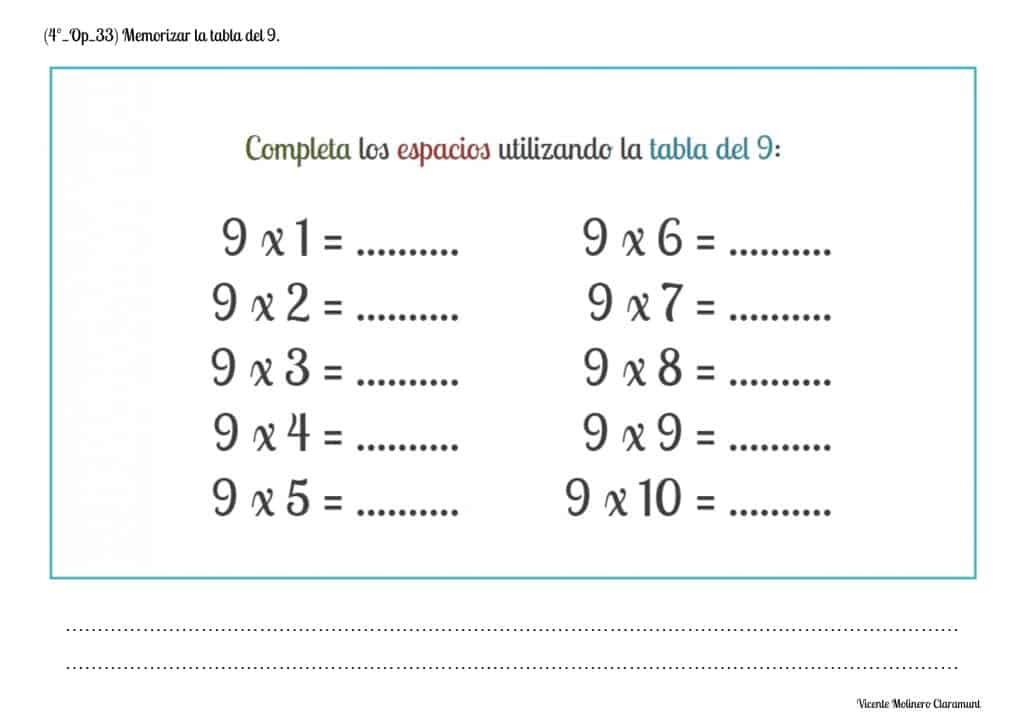#### Memorize and learn 10 Times Multiplication Table

The multiplication Table of 10 is another of the easiest to learn (along with the tables of 2 and 5) since the products are increased by 10 by 10 and therefore, the result will always end in 0 in the ones digit.

We can take advantage of their learning to introduce the concept of "decuple a number" throughout the learning of the table of 10 since the result of each multiplication will always be ten times the multiplier digit (for example, 7 x 10 = 70, where 70 equals ten times 7).

A simple strategy that we can use to learn the 10 times table fluently is to add ten ones to the result of the previous multiplication progressively.

SPANISHENGLISH10 x 0 = 0

10 x 1 = 10 = 10

10 x 2 = 10 + 10 = 20

10 x 3 = 10 + 10 + 10 = 30

10 x 4 = 10 + 10 + 10 + 10 = 40

10 x 5 = 10 + 10 + 10 + 10 + 10 = 50

10 x 6 = 10 + 10 + 10 + 10 + 10 + 10 = 60

10 x 7 = 10 + 10 + 10 + 10 + 10 + 10 + 10 = 70

10 x 8 = 10 + 10 + 10 + 10 + 10 + 10 + 10 + 10 = 80

10 x 9 = 10 + 10 + 10 + 10 + 10 + 10 + 10 + 10 + 10 = 90

10 x 10 = 10 + 10 + 10 + 10 + 10 + 10 + 10 + 10 + 10 + 10 = 100

#### Compare the multiplication tables of 2, 4 and 8, establishing relationships between them

After reviewing and learning the multiplication tables from 1 to 10, we provide you with educational worksheets ready to download and print in PDF format so that you can assimilate and develop the concepts double and half by relating tables 2, 4 and 8.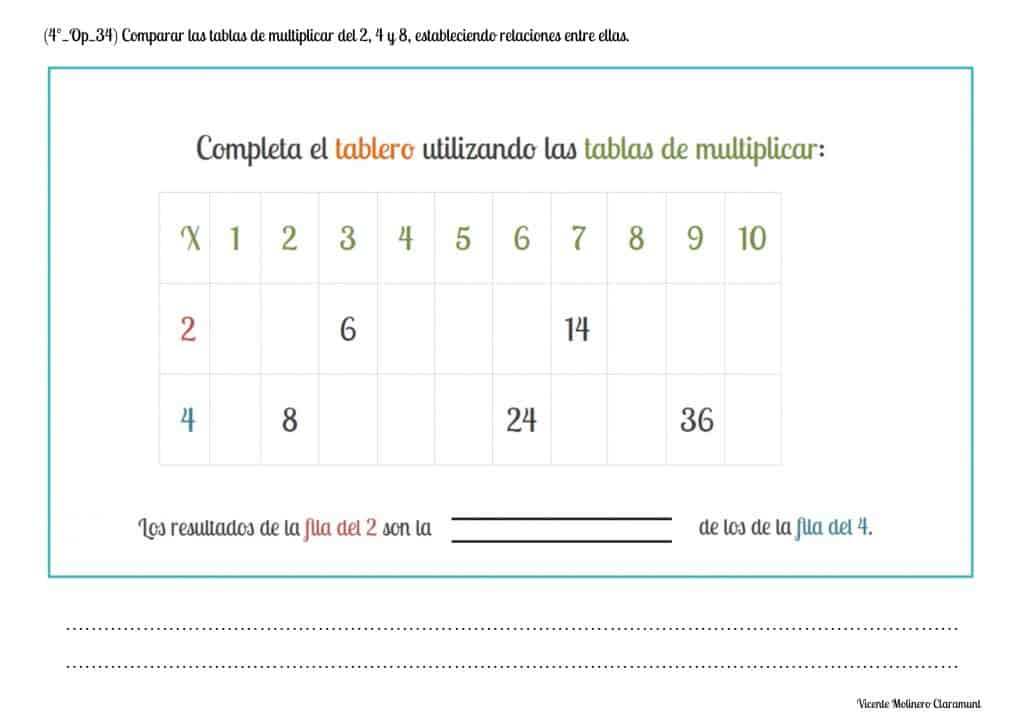#### Compare the multiplication tables of 3, 6 and 9, establishing relationships between them

We propose some didactic exercises so that you can correctly interpret the concepts of double, triple, half and third when relating the tables of 3, 6 and 9.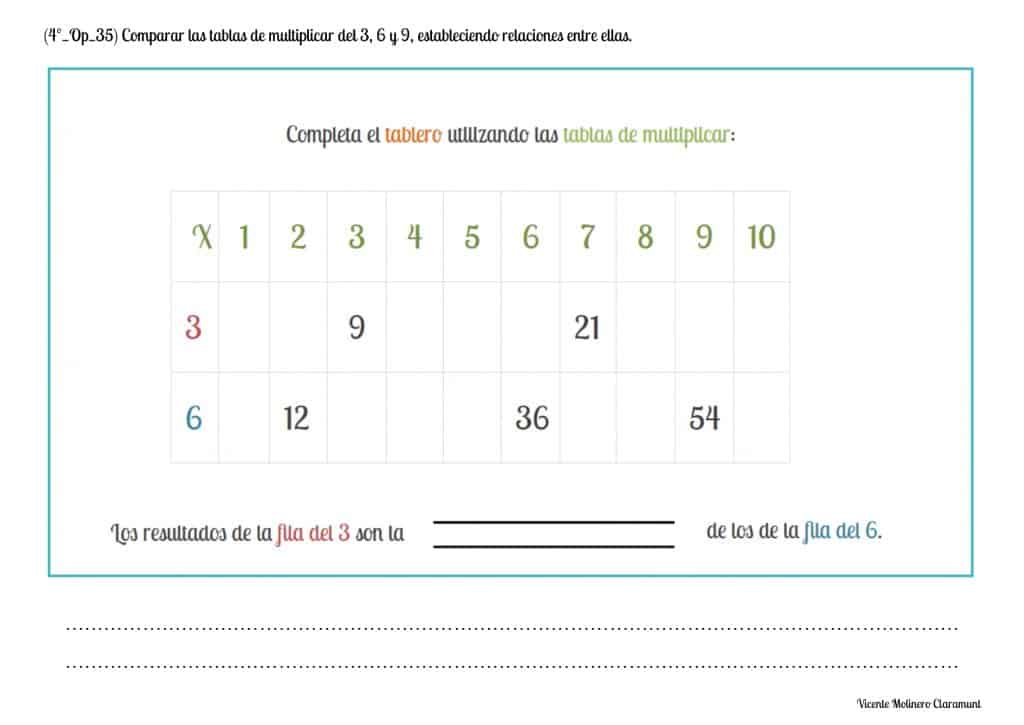#### Compare the multiplication tables of 5 and 10, establishing relationships between them

We offer you different activities so that you can understand step by step the concepts of double and half by relating the times tables of 5 and 10 using a visual board.Select more exercises, worksheets and activities of Mathematics for each of the 4 learning blocks of the Educational Curriculum ("Numbers and Operations", "Quantities and Measurements", "Geometric and Spatial Reasoning" and "Data Analysis and Probability") aimed at improving the logical-mathematical competencies and skills that are developed throughout "Grade 4" or "Year 5":